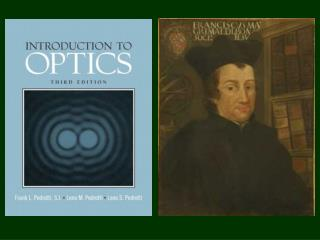DownloadDownload Presentations sin q

# s sin q

Télécharger la présentation## s sin q

- - - - - - - - - - - - - - - - - - - - - - - - - - - E N D - - - - - - - - - - - - - - - - - - - - - - - - - - -
##### Presentation Transcript

1. b 1 0.8 0.6 0.4 0.2 0 -8 -4 0 4 8 Diffraction from Apertures s sinq ds s r

2. r dA(x,y,z) r R r y x z field amplitude per unit area Phase differences in r’s Any Aperture P(X,Y,Z) a b

3. They are close enough for the amplitude term (the difference is small compared to the screen distance) They are not close enough for the phase term (the difference is not small compared to the wavelength)

4. binomial expansion:

5. For any shape! Rectangular:

6. From here its just like the single slit:

7. Circular Apertures D = s sinq s q R P

8. f F Circular Apertures P q r R

9. F is arbitrary, might as well make it zero: Bessel Function!

10. Bessel function of the first kind (J) of order zero (0) Bessel functions Jm(x) solve this differential equation: Recurrence relations let you solve integrals and derivatives: They can be expressed as series: